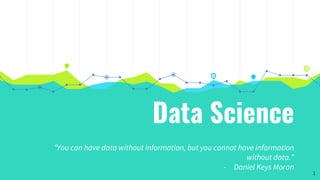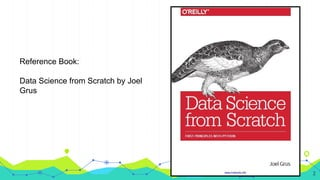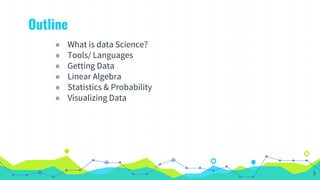Se está descargando tu SlideShare. ×

# Introduction to data science

Anuncio
Anuncio
Anuncio
Anuncio
Anuncio
Anuncio
Anuncio
Anuncio
Anuncio
Anuncio
AnuncioCargando en…3
×

1 de 36 Anuncio

# Introduction to data science

This is a presentation prepared on Introduction to data science for the fulfillment of an university assignment

This is a presentation prepared on Introduction to data science for the fulfillment of an university assignment

Anuncio
Anuncio

### Introduction to data science

1. 1. Data Science “You can have data without information, but you cannot have information without data.” - Daniel Keys Moran 1
2. 2. Reference Book: Data Science from Scratch by Joel Grus 2
3. 3. Outline ◉ What is data Science? ◉ Tools/ Languages ◉ Getting Data ◉ Linear Algebra ◉ Statistics & Probability ◉ Visualizing Data 3
4. 4. 1.What is Data Science? “Data! Data! Data!” he cried impatiently. “I can’t make bricks without clay.” —Arthur Conan Doyle 4
5. 5. Hacking Skills Math and Statistics Knowledge Substantive expertise Data Science 5
6. 6. ❖ “someone who knows more statistics than a computer scientist and more computer science than a statistician” ❖ Someone who extracts insights from messy data Data Scientist? 6
7. 7. 7
8. 8. 8
9. 9. 2. Tools / Languages People are still crazy about Python after twenty-five years, which I find hard to believe. —Michael Palin 9
10. 10. Tools / Languages ❖ R ❖ Python ❖ Matlab ❖ SQL ❖ Excel ❖ Java ❖ SAS (Statistical Analysis System) ❖ SPSS (Modeler and Analytics) ❖ Hadoop (File System Computing) 10
11. 11. Python ❖ Easy ❖ Python 2.7 ❖ Different Libraries for Data mining Numpy SciPy Pandas Matplotlib Scikit-learn 11
12. 12. 3. Getting Data To write it, it took three months; to conceive it, three minutes; to collect the data in it, all my life. —F. Scott Fitzgerald 12
13. 13. Different ways of getting data ◉ stdin and stdout ◉ Reading files ◉ Scraping the web ◉ Using APIs 13
14. 14. Using Twitter API ◉ Python 2.7 ◉ Python- Twitter libraries (Birdy, TwitterAPI, Twitter search, Twython) ◉ Twython Pip install twython ◉ Go to https://apps.twitter.com/. ◉ Click Create New App. ◉ Click “Create my access token.” ◉ Run SearchAPI.py 14
15. 15. 4. Linear Algebra Is there anything more useless or less useful than Algebra? —Billy Connolly 15
16. 16. Vectors ❖ Vectors are points in some finite-dimensional space ❖ A good way to represent numeric data ❖ Simplest from-scratch approach is to represent vectors as lists of numbers Ex :- If you have the heights, weights, and ages of a large number of people, you can treat your data as three-dimensional vectors (height, weight, age) 16
17. 17. Matrices ❖ A matrix is a two-dimensional collection of numbers. ❖ We can represent matrices as lists of lists ❖ We can use a matrix to represent a data set consisting of multiple vectors Ex :- If you had the heights, weights, and ages of 1,000 people you could put them in a 1 000 × 3 matrix 17
18. 18. Linear Algebra + Data Science To extract useful information from large, often unstructured, sets of data, in some data mining applications huge matrices are used. Ex :- The task of extracting information from all Web pages available on the Internet is done by search engines. The core of the Google search engine is a matrix computation 18
19. 19. 19
20. 20. 20
21. 21. 21
22. 22. 5. Statistics & Probability 22
23. 23. Statistics Statistics refers to the mathematics and techniques with which we understand data. Mean Median Range Variance Standard Deviation……... 23
24. 24. Statistics Framing questions statistically allow us to leverage data resources to extract knowledge & obtain better answers. A statistical framework allows researchers to distinguish between causation & correlation , thus to identify interventions that will cause changes in outcomes To establish methods for prediction & estimation to quantify their degree of certainty 24
25. 25. Probability Hard to do data science without some sort of understanding of probability and its mathematics. Conditional Probability Bayes’s Theorem Random Variables Continuous Distributions Normal Distribution……….. In an uncertain world, it can be of immense help to know and understand chances of various events. You can plan things accordingly. 25
26. 26. 6.Visualizing Data I believe that visualization is one of the most powerful means of achieving personal goals. —Harvey Mackay 26
27. 27. Brain receives 8.96 Megabits of data from the eye every second. Average person comprehends 120 words per minute reading Visual Comprehension speed Reading Comprehension speed 27
28. 28. 28
29. 29. Why Visualization? ❖ A fundamental part of the data scientist’s toolkit is data visualization. ❖ To explore data ❖ To communicate data 29
30. 30. 30
31. 31. Current Examples A Day in the life,NYC Taxis http://chriswhong.github.io/nyctaxi/ U.S.Gun Deaths in 2013 http://www.guns.periscopic.com/?year=2013 31
32. 32. Tools for Data Visualization ❖ Matplotlib ❖ Seaborn ❖ D3.js ❖ Bokeh ❖ Ggplot ❖ R 32
33. 33. Example with R ◉ Iris data set ◉ Iris is a data frame with 150 cases (rows) and 5 variables (columns) named Sepal.Length, Sepal.Width, Petal.Length, Petal.Width, and Species. 33
34. 34. data(iris) iris summary(iris) summary(iris\$Petal.Length) barplot(iris\$Petal.Length) #Creating simple Bar Graph 34
35. 35. plot(x=iris\$Petal.Length) # Creating scatter plot plot(iris\$Petal.Length, iris\$Petal.Width, pch=c(23,24,25)[unclass(iris\$Species)], main=" Iris Data") plot(iris\$Petal.Length, iris\$Petal.Width, pch=21, bg=c("red","green3","blue")[unclass(iris\$Species)], main="Iris Data") pairs(iris[1:4], main = " Iris Data", pch = 21, bg = c("red", "green3", "blue")[unclass(iris\$Species)]) 35
36. 36. 36